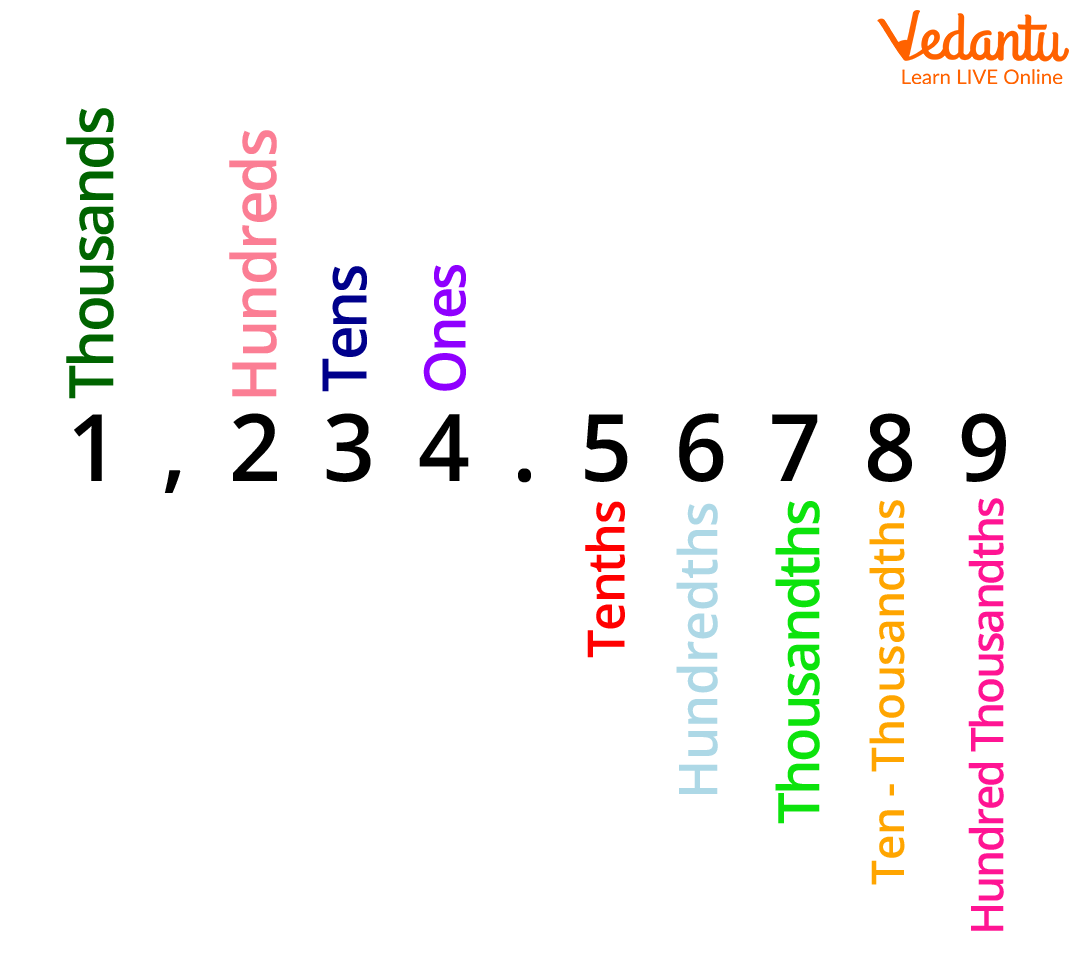Courses
Courses for Kids
Free study material
Offline Centres
More

# Understanding Tenths with Solved ExamplesLast updated date: 06th Dec 2023
Total views: 161.7k
Views today: 2.61k## What are Tenths?

Sheila loves to collect a list of numbers from 1 to 9. One day, she bought nine containers and started numbering each from 1 to 9. At the bottom of the 9th container, she found something looking like ‘0’. She saw this for the first time and wondered what I could do with ‘0’? Well, she first placed ‘0’ after ‘1’ to make it 10, then after ‘2’ to make it ‘20’. In this way, she kept on making a two-digit number by placing zero after each digit.

Now, we notice that ‘10’ resembles our main topic Tenths, so what are tenths? Well, the definition of tenths says that it is the first digit to the right of the decimal point; one out of 10 equal parts of a whole.

Now, let us understand what tenths are along with certain examples that we will cover on this page.

## Examples of Tenths

These are the following values that we know so far:

• Ones

• Tens

• Hundreds

• Thousands

• Ten-thousands

• Hundred-thousands, etc.

Let us say we have a big number and the position of each number is determined by the number of zeroes it has after itself. Assume that the number is 897.625 and find the place value of each digit.

• 7 is at unit’s place, so 7 * 1 = 7.

• 9 has a place value of tens, i.e., 10, so 9 * 10 = 90.

• 8 has a place value of thousands or 1000, so 8 * 1000 = 8000.

Now, there comes another three-digit number after a decimal, which we need to find the place value of. Do you know that there is a difference in finding the place value of the numbers after the decimal? Well, there is! So, let us understand the process.

Please note that the value to the right of the decimal point is always a fraction of a value less than 1. So, the positions of a three-digit number mentioned in the above example are determined by the following position holders:

• Tenths

• Hundredths

• Thousandths

• Ten-thousandths

• Hundred-thousandths, and so on

Now, let us see for .625

• 6 has a value of 6/10th

• 2 has a value of 2/100th

• 5 has a value of 5/1000th

We notice that the position for tenths is calculated by dividing the digit just after the decimal point by 10.Now, let us take another example of 778613.962.

• 7 has a place value of 7 * 100000 = 700,000.

• 7 has a value of  7 * 10000 = 70,000.

• 8 has a value of 8 * 1000  = 8,000.

• 6 has a value of 6 * 100 = 600.

• 1 has a value of 1 * 10 = 10.

• 3 has a value of 3.

Similarly, the place value of .962 is calculated as:

• 9 has a place value of 9/10th (tenth),

• 6 has a place value of 6/100th, and

• 2 has a place value of 2/100th.

So, this is how we can determine the tenth place value of a two-digit, three-digit, or four-digit number, and so on. The position of the tenth is 1/10th of the digit just right after the decimal point.

## FAQs on Understanding Tenths with Solved Examples

1. What are tenths in decimals?

Decimal numbers are equal to fractions and they are less than 1. For example, suppose there are 5 pieces of chocolates in a box and they are numbered 12345. So, here, the position of 2 will be 2/10th, 3 will be 3/10th, 4 will be 4/10th, and so on till 5/10000th.

We can simply write 2 * 0.1, 3 * 0.01, 4 * 0.01, and so on if we are considering decimal points here.

2. What is a fraction of a tenth and determine the number of tens in numbers 20 and 100?

The fraction of a tenth can be written as 1/10 and in a percentage form, we write it as 10% of a digit. For example, the tenth place value of 2 is 2/10 or 2 * 0.1 = 0.2.

The number of tens in 20 is 1, i.e., 2 * 10 = 20 and the number of tens in 100 is 2, i.e., 10 * 10, i.e., 2 tens.